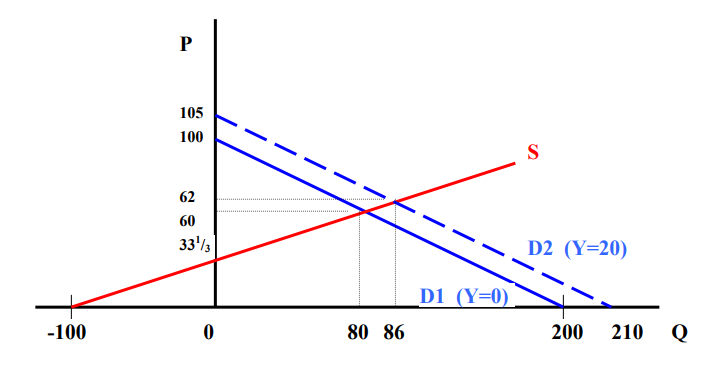In: Economics

# Given the above Demand and Supply functions, what is the impact on the Market Equilibrium of Y increasing from 0 to 20?

QD = 200 -2P + ½Y

QS = 3P – 100

Given the above Demand and Supply functions, what is the impact on the Market Equilibrium of Y increasing from 0 to 20?

## Solutions

##### Expert Solution

QD = QS

200 -2P + ½Y = 3P – 100 5P

= 300 + ½Y P = 60 + 1 /10Y

Knowing P, find Q

Q = 3(60 + 1 /10Y) -100

= 80 + 3 /10Y

As Y increases P increases by 1/10 of the increase in Y, and Q increases by 3/10 of Y increase.

Equilibrium P and Q when Y = 20

P = 60 + 1 /10Y

P = 60 + 1 /10(20) = 62 that is, increase in P by 1/10 of 20 = 2

Q = 80 + 3 /10Y

Q = 80 + 3 /10(20) = 86 that is, increase in Q by 3 /10 of 20 = 6

Drawing the graph

Intercepts:

Supply curve

(Q, P): (-100, 0) and (0, 331 /3 )

Demand curve

Y=0:

D1 (Q,P): (200, 0) and (0, 100)

Y=20:

D2 (Q,P): (210, 0) and (0, 105)Increase in income increases the equilibrium price and quantity demanded increases.